Nanomedicine, Volume I: Basic Capabilities

Robert A. Freitas Jr., Nanomedicine, Volume I: Basic Capabilities, Landes Bioscience, Georgetown, TX, 1999

Two types of electrostatic forces may act to hold particles to surfaces. The first type of force is due to bulk excess charges present on the particle or surface which produce a classical Coulombic attraction known as the electrostatic image force. Consider two spheres of radii r1 and r2 located a distance zsep apart, where zsep << r1, r2. Then following Eqn. 4.40 the image force is given by:1148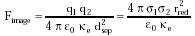{Eqn. 9.9}

where q1 and q2 are charge on the two spheres (coul), s1 and s2 are the surface charge densities (coul/m2), e0 = 8.85 x 10-12 farad/m (permittivity constant), ke is the dielectric constant of the medium, and dsep ~ r1 + r2 is the distance between charge centers. Taking s1 ~ s2 = 10-5 coul/m2 (~63 charges/micron2; cf. ~1000 charges/micron2 for "highly charged surfaces"1151), r1 ~ r2 = 0.5 micron and ke = 1 in vacuo, then Fimage ~ 0.009 nN.

The electrostatic image force between two flat plates of equal interfacial area A, equal charge density s, and uniform separation zsep with zsep << A1/2, is:727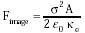{Eqn. 9.10}

For s ~ 10-5 coul/m2 and A = 1 micron2 in vacuo (ke = 1), Fimage ~ 0.006 nN (~0.00006 atm). At larger zsep and a voltage differential Vplate between the plates with capacitance Cplate, then: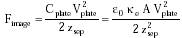{Eqn. 9.11}

Thus if A = 1 micron2, Vplate = 1 volt, and zsep = 0.2 micron, then Fimage ~ 0.1 nN.

The second and more important type of electrostatic force for very small particles is the electrostatic contact potentials, also known as induced electrical double layer forces.1146 When two different initially uncharged materials with different local energy states and work functions are brought into contact, charge flows between them until an equilibrium is reached. The resulting potential difference is called a contact potential, Vcontact, which typically ranges from ~0-1 volt. In the case of two metals, only the surface layer which is xcontact ~ 1 nm thick (the gap for tunneling) carries contact charges; for semiconductors and insulators, these regions may extend into the bulk up to xcontact ~ 1 micron or deeper.1146 For a spherical particle of radius r at a distance zsep from a surface, the induced charge density1147 is s = e0 Vcontact / zsep and the contact force is:1146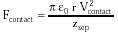{Eqn. 9.12}

Taking r = 0.5 microns, Vcontact = 0.5 volts, and zsep = 5 nm, then Fcontact ~ 1 nN (~0.01 atm). Induced charge densities vary experimentally from 2 x 10-7 coul/m2 (~2 x 10-11 atm contact pressure) for polystyrene on polystyrene1153 up to 2 x 10-2 coul/m2 (~200 atm) for SiO2 on mica.1154 In this latter study, a pair of contact-adhered silica/silica sheets required a force of ~0.08 nN/nm to pull them apart and a mica/mica pair required ~ 0.11 nN/nm; however, due to contact electrification a silica/mica pair required 6.6-8.8 nN/nm to separate.1154 Proper nanomechanical design can avoid or enhance these effects, as required.10

Double layer electrostatic contact forces usually predominate over electrostatic image forces for small particles; image forces are important only for materials that can carry high surface charges, such as polymers of poor conductivity or other extreme insulators. Because of their r/zsep dependency, contact electrical forces are usually more important than van der Waals forces only for large particles and at separations wider than ~100 nm, although electrical forces can dominate in smaller particles if the surface asperities of the particles are significant enough to remove the bulk of the particle from the contact point, thus greatly reducing the van der Waals interaction.1146 In either case, the total forces of adhesion far outweigh the force of gravity, given by: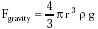{Eqn. 9.13}

For density r = 3510 kg/m3 for diamond, acceleration of gravity g = 9.81 m/sec2, and particle radius r = 0.5 micron, Fgravity ~ 10-5 nN; for r = 1 nm, Fgravity = 10-13 nN.

Entropic and electrostatic effects can sometimes interact to produce an apparent repulsion between opposite charges -- as, for example, between positively charged bilayer surface and negatively charged colloidal particles.3674

Last updated on 20 February 2003